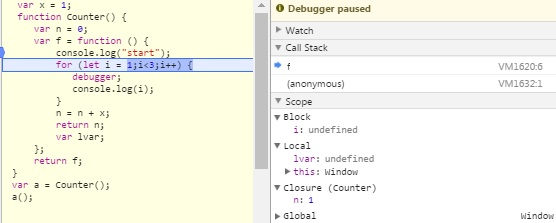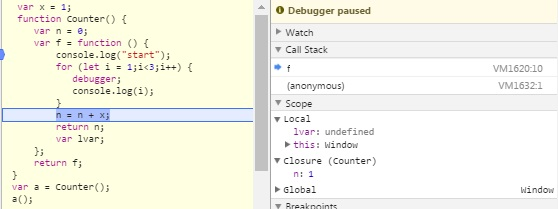# 作用域和闭包

## 概述

• 静态作用域
指标识符的作用范围是由标识符的声明位置和程序的结构决定的，也就是说某段代码所参照的标识符是在哪定义的，通过对程序代码进行词法解析即可确定，标识符的作用域是静态不变的。
• 动态作用域
指标识符的作用范围是由程序运行时函数的调用情况决定的，也就是说某段代码所参照的标识符是在哪定义的，需在程序运行时根据执行栈才能确定，标识符的作用域可能会是动态变化的。

```var i = 1,
f1 = function() {
i = i+1;
console.log(i);
},
f2 = function() {
var i = 2;
f1();
f1();
};
f3 = function() {
f1();
f1();
};
f2();
f3();```

• f2()
• 第一次调用的 f1()
将全局变量 i 由1变为 2 ， 并输出2
• 第二次调用的 f1()
将全局变量 i 由2变为 3 ，并输出 3
• f3()
• 第一次调用的 f1()
将全局变量 i 由3变为 4，并输出 4
• 第二次调用的 f1()
将全局变量 i 由4变为 5，并输出 5

1. 被 f2() 调用的时候
f2() 里也有同名的 i 变量，由于排在全局变量 i 的前面，这时 f1() 操作的是 f2() 里的局部变量 i。
2. 被 f3() 调用的时候
f3() 里没有局部变量 i，因此直接操作的是全局变量 i。

• f2()
• 第一次调用的 f1()
将 f2() 的局部变量 i 由2变为 3，并输出 3
• 第二次调用的 f1()
将 f2() 的局部变量 i 由3变为 4，并输出 4
• f3()
• 第一次调用的 f1()
将全局变量 i 由 1 变为 2，并输出 2
• 第二次调用的 f1()
将全局变量 i 由 2 变为 3，并输出 3

## 作用域的类型

1. 全局作用域 （global scope）
全局作用域里的变量称为全局变量，所有程序都能访问。
大部分语言都支持全局作用域，既有象 Basic 一样的只有全局作用域的语言，也存在象 Python 这样不让程序简单的就能修改全局变量的语言。
JavaScript 支持全局作用域
2. 文件作用域 （file scope）
文件作用域与全局作用域类似，但变量只能是同一个源文件模块里的程序才能访问。
支持文件作用域的语言比较少，有C/C++等。
JavaScript 不存在文件作用域
3. 函数作用域 （function scope）
函数作用域是一个局部作用域，变量在函数内声明，只在函数内部可见。大部分语言都支持函数作用域。
JavaScript 支持函数作用域
4. 代码块作用域 （block scope）
代码块作用域也是一个局部作用域，变量在代码块 ({}) 内声明，只在代码块内部可见。
支持代码块作用域的有 C/C++、C#、Java。
JavaScript 从 ES6 开始支持代码块作用域
5. 静态局部作用域 （static local scope）
静态局部作用域也是函数内部的局部作用域，其特殊性是即使函数执行结束后变量也不会被释放，每次函数代码的执行访问的都是同一个变量。
支持静态局部作用域的语言比较少，基本上都是一些历史比较悠久的语言，如 C/C++、Fortran 等。
JavaScript 不存在静态局部作用域
6. 闭包作用域（closure scope）
闭包是一种让函数的代码能够访问函数声明（函数对象被创建）时的作用域内（上下文环境）的变量机制。闭包在函数式语言中非常普遍。
JavaScript 支持闭包作用域

## 局部作用域

### 函数作用域

```function hi() {
for (var i = 0; i < 10; i++) {
var value = "hi " + i ;
}
console.log(i); // 输出:10
console.log(value);//输出 : hi 9
}
hi();```

### 代码块作用域

ECMAScript6 之后，函数内用 let 声明的变量和 const 声明的常量都属于代码块作用域。

```function hi() {
for (let i = 0; i < 10; i++) {
let value = "hi " + i ;
}
try {
console.log(i);  // Uncaught ReferenceError: i is not defined
} catch (e){
console.log(e.toString());
}
try {
console.log(value);// Uncaught ReferenceError: value is not defined
} catch (e){
console.log(e.toString());
}
}
hi();```

### 闭包作用域

1. 闭包是将函数定义时的局部作用域环境保存起来后生成的一个实体。
2. 闭包实现了一个作用域，函数始终是运行在它们被定义的闭包作用域里，而不是它们被调用的作用域里。
3. 闭包可以嵌套，全局作用域→闭包(0..n)作用域→函数作用域→代码块(0..n)作用域就整个的形成了一个代码执行时的作用域链。

```  var x = 1;
function Counter() {
var n = 0;
var f = function () {
n = n + x;
return n;
};
return f;
}```

`var a = Counter();`

Counter()执行后的环境状态如下：

```  ┌────────────────┐
全局环境             ┌────────────────────────────────┐
x => 1         ←─  Counter()执行时的局部环境(a的闭包)     ←─ a();
a => function           n => 0                          ←─ a();
└────────────────────────────────┘
└────────────────┘   ```

```console.log("a()=" + a());  //输出： a()=1
console.log("a()=" + a());  //输出： a()=2
console.log("a()=" + a());  //输出： a()=3
console.log("a()=" + a());  //输出： a()=4```

```  var a = Counter();
var b = Counter();```

Counter()两次执行后的环境状态如下：

```┌──────────────────┐       ┌───────────────────────────────────────┐
全局环境           ←─    Counter()第1次执行时的局部环境(a的闭包)     ←─ a();
x => 1                      n => 0                             ←─ a();
a => function        └───────────────────────────────────────┘
b => function        ┌───────────────────────────────────────┐
←─     Counter()第2次执行时的局部环境(b的闭包)     ←─ b();
n => 0                             ←─ b();
└───────────────────────────────────────┘
└──────────────────┘                          ```
```
console.log("a()=" + a()); // 输出：a()=1
console.log("a()=" + a()); // 输出：a()=2
console.log("b()=" + b()); // 输出：b()=1
console.log("a()=" + a()); // 输出：a()=3
console.log("b()=" + b()); // 输出：b()=2```

## 作用域链

JavaScript 中的作用域链有两种: 一种是函数创建时保存在函数对象属性中的、静态存在的作用域链,还有一种是程序执行时，与执行上下文相关联的、动态存在的作用域链,下面对这两种作用域链分别进行说明。

### 执行上下文的作用域链

（这里以函数的执行为例进行说明，与函数的执行相比，全局代码执行时的作用域链更为简单，没有函数作用域和闭包作用域。）

ES6 之后支持代码块作用域，如果代码块里存在 let 定义的变量，即会出现作用域延长的现象。ES5 之前也有两种特殊情况下会发生这种现象：

• try-catch 语句中的 catch 块
• with 语句

```  var x = 1;
function Counter() {
var n = 0;
var f = function () {
console.log("start");
for (let i = 1;i<3;i++) {
debugger;
console.log(i);
}
n = n + x;
return n;
var lvar;
};
return f;
}
var a = Counter();
a();```

1. 进入代码块
一个新的 block (块作用域)被压至作用域链的顶端2. 退出代码块
block (块作用域)被弹出， 作用域链顶端恢复为 local (函数作用域)